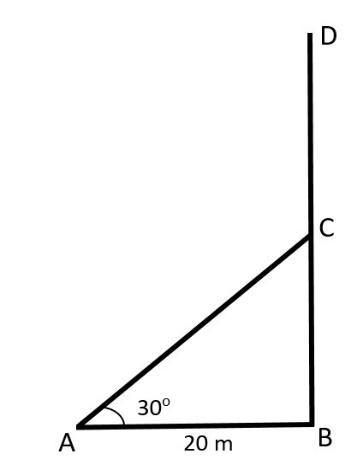Courses
Courses for Kids
Free study material
Free LIVE classes
MoreLIVE
Join Vedantu’s FREE Mastercalss

# The upper part of a tree, broken by wind in two parts, makes an angle of ${{30}^{\circ }}$ with the ground. The top of the tree touches the ground at a distance of 20 metres from the foot of the tree. Find the height of the tree before it is broken.Verified
361.8k+ views
Hint: Here when the tree is broken, a triangle is formed and using the triangle we can find the height of the tree.

First with the given data we have to draw the figure.In this figure BD is the tree, due to wind it is broken from the point C where $CD=CA$. The broken tree makes an angle of ${{30}^{\circ }}$ with the ground. i.e. $\angle BAC={{30}^{\circ }}$
Here, the distance between the top of the tree that touches the ground and foot of the tree is 20 m. i.e. $AB=20\text{ m}$.
Here, we have to calculate the height of the tree, i.e. $BD$.
From the figure, we can say that,
\begin{align} & BD=BC+CD \\ & BD=BC+CA \\ \end{align}
For that, first consider $\vartriangle ABC$, the tangent of A is given by,
\begin{align} & \tan {{30}^{\circ }}=\dfrac{oposite\text{ }side}{adjacent\text{ }side} \\ & \tan {{30}^{\circ }}=\dfrac{BC}{AB} \\ & \tan {{30}^{\circ }}=\dfrac{BC}{20}\text{ }....\text{ (1)} \\ \end{align}
We know that $\tan {{30}^{\circ }}=\dfrac{1}{\sqrt{3}}$, by substituting this value in equation (1) we get:
$\dfrac{1}{\sqrt{3}}=\dfrac{BC}{20}$
By cross multiplication we get,
\begin{align} & 20=\sqrt{3}BC \\ & BC=\dfrac{20}{\sqrt{3}} \\ \end{align}
To find $CA$, consider the cosine of A, we obtain:

\begin{align} & \cos {{30}^{\circ }}=\dfrac{\text{adjacent }side}{hypotenuse} \\ & \cos {{30}^{\circ }}=\dfrac{AB}{CA} \\ & \cos {{30}^{\circ }}=\dfrac{20}{CA}\text{ }.....\text{ (2)} \\ \end{align}
We also know that $\cos {{30}^{\circ }}=\dfrac{\sqrt{3}}{2}$ our equation (2) becomes:
$\dfrac{\sqrt{3}}{2}=\dfrac{20}{CA}$
By cross multiplication we get,
\begin{align} & \sqrt{3}CA=2\times 20 \\ & \sqrt{3}CA=40 \\ & CA=\dfrac{40}{\sqrt{3}} \\ \end{align}
Next, we have to calculate $BD$ where.
\begin{align} & BD=BC+CA \\ & BD=\dfrac{20}{\sqrt{3}}+\dfrac{40}{\sqrt{3}} \\ \end{align}
By taking the LCM we get:
\begin{align} & BD=\dfrac{20+40}{\sqrt{3}} \\ & BD=\dfrac{60}{\sqrt{3}} \\ & BD=\dfrac{60}{1.73}\text{ }....\text{(}\sqrt{3}=1.73) \\ & BD=34.682 \\ \end{align}
Hence, we obtain the height of the tree is $34.682$.

Note: Here, you may get confused regarding the tree and the broken part of the tree, from the figure we can say that CA is the broken part of the tree and CA=CD.
Last updated date: 30th Sep 2023
Total views: 361.8k
Views today: 3.61k